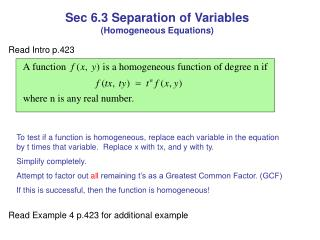DownloadDownload PresentationSec 6.3 Separation of Variables (Homogeneous Equations)

# Sec 6.3 Separation of Variables (Homogeneous Equations)

Download Presentation## Sec 6.3 Separation of Variables (Homogeneous Equations)

- - - - - - - - - - - - - - - - - - - - - - - - - - - E N D - - - - - - - - - - - - - - - - - - - - - - - - - - -
##### Presentation Transcript

1. Sec 6.3 Separation of Variables (Homogeneous Equations) Read Intro p.423 To test if a function is homogeneous, replace each variable in the equation by t times that variable. Replace x with tx, and y with ty. Simplify completely. Attempt to factor out all remaining t’s as a Greatest Common Factor. (GCF) If this is successful, then the function is homogeneous! Read Example 4 p.423 for additional example

2. This is f(x,y) This is f(x,y) This function is homogeneous of degree 2. This function is NOT homogeneous since I cannot factor t’s out due to the 1!

3. If a differential equation is homogeneous then it can be transformed into a differential equation whose variables are separable by the substitutiony=vxwhere v is a differentiable function of x. Note: if y = vx, then dy = v dx + x dv